## research papersJOURNAL OFSYNCHROTRONRADIATION
ISSN: 1600-5775
Volume 25| Part 2| March 2018| Pages 378-384

## Qu­antitative investigation of linear arbitrary polarization in an APPLE-II undulator

aDiamond Light Source, Harwell Science and Innovation Campus, Didcot OX11 0DE, UK, and bInstitute of Precision Optical Engineering, Tongji University, Shanghai 200092, People's Republic of China
*Correspondence e-mail: hongchang.wang@diamond.ac.uk

(Received 2 November 2017; accepted 1 February 2018; online 22 February 2018)

Insertion devices are utilized at synchrotron radiation facilities around the world for their capability to provide a high-brilliance X-ray beam. APPLE-II type undulators are especially important for their capacity to switch between a variety of photon beam polarization states. A high-precision soft X-ray polarimeter has been used to investigate the polarization calibration of an APPLE-II undulator (period length λu = 64 mm) installed on beamline I06 at Diamond Light Source. Systematic measurement of the beam polarization state at a range of linear arbitrary angles has been compared with the expected result for a given set of undulator gap and row phase parameters calculated from theory. Determination of the corresponding Stokes–Poincaré parameters from the measured data reveals a discrepancy between the two. The limited number of energy/polarization combinations included in the undulator calibration tables necessitates the use of interpolated values for the missing points which is expected to contribute to the discrepancy. However, by modifying the orbit of the electron beam through the undulator by at least 160 µm it has been found that for certain linear polarizations the discrepancies can be corrected. Overall, it is suggested that complete correction of the Stokes–Poincaré parameters for all linear angles would require alteration of both these aspects.

### 1. Introduction

Undulator sources are commonly used on beamlines at modern synchrotron radiation facilities owing to their capacity to deliver brilliant soft X-ray beams with variable polarization (Sasaki, 1994; Hwang & Yeh, 1999; Weiss et al., 2001). Among the variety of undulator types available, APPLE-II helical undulators (HUs), consisting of four magnet array quadrants Q1–Q4, are widely used for their flexibility in varying the emitted radiation polarization between left-handed and right-handed circular polarization and linear polarization with arbitrary angle (which will be the focus here) by adjusting the relative position of the magnet arrays. In the latter case, the undulator parameters in question are the gap between the top and bottom array pairs, and the longitudinal offset (hereafter referred to as row phase') which for linear light is equal and opposite for diagonally opposing arrays, i.e. quadrants Q2 and Q4 as indicated in Fig. 2 (Longhi et al., 2013). Therefore, to set linear polarization with arbitrary angle we have a two-valued two-dimensional function F representing the beam state which must be invertible (for energy/angle readback) (Young et al., 2002),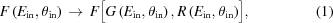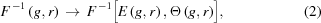where G and R are the forward transformations to determine gap g and row phase r from the input energy Ein and angle θin, and E and Θ are the reverse transformations to obtain the energy and angle readback values. Three-dimensional magnetostatics computer codes (Chubar et al., 1998) can be used to numerically calculate the magnetic field generated by a given gap and row phase configuration. Subsequently, the near-field synchrotron radiation emission from an electron passing through this generated magnetic field can be computed using an approach based on retarded potentials, and propagated by applying scalar diffraction theory via Fourier optics methods (Chubar & Elleaume, 1998). The polarization state of the emitted radiation is finally extracted from the propagated electric field. However, the inverse problem of determining the magnetic field, and hence the undulator parameters, to produce photons of a specified energy and polarization state is more challenging. A typical approach requires simulating a subset of the possible undulator configurations to predict the resulting photon energy and polarization state. This forms a look-up table which is used to select the appropriate gap and row phase for the input energy and angle. However, the simulated set of undulator parameters is sparse compared with the possible configurations and not evenly spaced in angle/energy, so interpolation of the results is necessary. An example of such a parameter set is shown in Fig. 1. The accuracy of the inverse transform from (gap, row phase) back to (energy, angle) is highly dependent on the density of the original grid of simulated undulator parameters, but simulation of all possible configurations is not feasible given the time-consuming nature of the computation. In the example given here, the linear arbitrary angles close to 0° (linear horizontal) and 90° (linear vertical) see large variation in the parameter values so calculation of the interpolated values in these regions is relatively less accurate. It is also unlikely that the state of the photon beam generated by a given set of undulator parameters will correspond precisely with that predicted by the theoretical calculation. Many factors related to the undulator can alter the polarization state which is observed at the endstation: inhomogeneity of the magnetic fields, small offsets in the magnet arrays, offsets in the electron beam trajectory, and off-axis alignment of the beamline acceptance. Other factors related to the beamline optics themselves may also contribute: steeper reflection angles (typically required for beamlines operating at energies below 100 eV), reflections in the energy range of the carbon edge due to carbon contamination of optics, manufacturing imperfections and misalignment may also impact the final polarization state.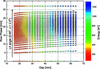Figure 1 Calculated undulator parameter set covering the complete range of linear arbitrary angles. These are linearly interpolated to produce look-up tables evenly spaced in energy and LA angle for compatibility with the underlying EPICS control system used to operate the undulator.

Here, we present measurements of the photon beam generated by an APPLE-II undulator acquired using a high-precision soft X-ray polarimeter. A wide range of polarization states from different undulator configurations were systematically characterized to better understand how the observed polarization state deviates from that predicated by theory. Additionally, manual alteration of the gap and row phase parameters away from the table values was investigated to determine how the beam characteristics may be improved. Finally, the effect of altering the electron orbit through the undulator on the polarization state was also investigated. Such information is useful for the future development of the beamline and its capacity to deliver a high-quality photon beam with a precisely defined polarization state.

### 2. Experimental details

The complete polarization measurements presented here were carried out on beamline I06 at Diamond Light Source, UK (Dhesi et al., 2010). A schematic of the experimental setup is shown in Fig. 2. Photons are produced by an identical pair of APPLE-II type HU-64 undulators. Each undulator has two diagonally opposing magnet arrays which are movable in order to set the row phase, while the other pair remains in a fixed position; the position of upper and lower movable arrays are referred to as top/bottom row phase (TRP/BRP), respectively. Photon energy is controlled over the range 70–2100 eV by altering the gap between the magnet arrays, and altering the relative phase of the two magnet rows changes the polarization of the beam. All the following polarization states may be selected: linear horizontal (LH), linear vertical (LV), linear at arbitrary angle (LA), left-handed circular (LC), right-handed circular (RC) and elliptical, although LC/RC and LV are only available above 106 eV and 130 eV, respectively. The third undulator harmonic is utilized for energies above 1300 eV. The gap and row phase are set completely independently for each device so prompt polarization switching, for example from LC to RC, is possible by changing from one undulator to the other with differing settings (Bahrdt et al., 2001; Quitmann et al., 2001; Schmidt & Zimoch, 2007). Alternatively, they may be operated together, in conjunction with a phasing device (single-period undulator) located between them, which allows for greater photon flux. Each undulator is 2.11 m in length, has 33 periods of period length λu = 64 mm and can achieve a minimum gap between the magnet rows of 15 mm. During the experiment, only one undulator was in use during any given measurement (primarily downstream); the gap of the other undulator and phasing unit remained at their maximum values so that they had no influence on the electron beam.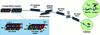Figure 2 Schematic layout of the experimental setup with the soft X-ray polarimeter installed on the beamline I06 branch line. With four magnet rows (quadrants Q1–Q4), the APPLE-II undulator offers the capability to alter the row phases of quadrants Q2 and Q4 independently, referred to as top and bottom row phase (TRP/BRP) here, along with the gap between the two magnet row pairs. This provides the capability of selecting almost any polarization state across the entire energy range of the source.

I06 consists of two branches which utilize a collimated plane-grating-monochromator (PGM) optical scheme (Follath & Senf, 1997). The measurements presented here were carried out on the branch line. The photon beam is collimated vertically (dispersion plane) by a cylindrical mirror before it passes through the PGM which contains 150 lines mm−1 (used here), 400 lines mm−1 and 1200 lines mm−1 gratings for low-energy, high-energy and high-resolution measurements, respectively. A toroidal mirror downstream of the PGM focuses the beam to the exit slit. A second toroidal mirror is used for re-focusing to a user-provided endstation. All the beamline mirrors and plane gratings have gold coating. Here, the grazing angles of incidence are sufficiently small that the polarization effect upon reflection may be neglected: the ratio of s- and p-polarization, determined using the reflectivity calculation software REFLEC (Schäfers, 2008), is Rs/Rp ≃ 1.006% for all the mirrors and Rs/Rp ≃ 1.012% for the grating at 375 eV.

The high-precision Diamond polarimeter employed to carry out these measurements is a multilayer-based system containing a transmission phase retarder and reflection analyzer (Wang et al., 2011). These are mounted in two azimuthally rotating stages which allow the retarder (α) and analyser (β) to rotate independently about the optical axis of the beam. Each azimuthal stage has an additional rotary stage which allows the multilayer incidence angles (retarder θR, analyser θA) to be tuned to the relevant Bragg angles. A photodiode is used to measure the light reflected from the analyser and is itself mounted on a rotating arm whose angle θD with respect to the optical axis is usually set to twice the analyser tilt angle. The mechanics all lie within a vacuum vessel that sits atop a hexapod providing six degrees of freedom for alignment. Prior to measurement with X-rays, the polarimeter is coarsely positioned and pre-aligned to the beam axis using externally mounted fiducial markers for reference with a laser tracker. Following this, fine alignment is achieved using X-rays. Low-level instrument control is performed via an Experimental Physics and Industrial Control System (EPICS) interface (Dalesio et al., 1994). However, the user interface and high-level control is provided by the Generic Data Acquisition (GDA) software (Enderby & Pulford, 2004; Gibbons, 2008). This is the standard data acquisition interface at Diamond Light Source and allows for all the scanning to be performed via Python scripts.

A single polarization measurement is normally carried out by detecting the light intensity incident upon the photodiode at many α and β angles, for example every 10°, covering a full 360° rotation of each axis. To reduce the total acquisition time, only eight positions (every 45°) are currently measured for one of the rotations, usually α, as this is sufficient for fitting. However, the future introduction of fly scanning, where data are recorded during the motor movement, will allow a much greater number of angular positions to be sampled with no sacrifice in acquisition time. To minimize the impact of angular misalignment on the fitting results, the data for opposing angles of this rotation (0 and 180°, 90 and 270°, etc.) are averaged since they are equivalent. Furthermore, since each half of the complete 360° rotation are equivalent, the acquisition time can be further reduced by another factor of two by limiting the measurement range of β to 0–180°. Finally, the scanning direction of each motion is optimized so no time is wasted returning to the start point each time. Through these optimizations, a complete measurement of a single polarization can be completed in approximately 15 min. A standard automatic alignment procedure was used to provide consistent angular alignment below 50 µrad before measurements were carried out.

At each combination of α and β the normalized intensity is characteristic of the photon polarization state as described by the theoretical calculation (Wang et al., 2012). By fitting this equation to the measured data, the so-called Stokes–Poincaré parameters P1, P2 and P3 can be extracted. These parameters can take values from −1 to 1 and describe the contribution of linear, linear at 45° and circular components to the overall polarization. Together, these parameters completely describe the polarization state of the light. Previously, this analysis was carried out using a tool developed in Igor Pro 6.32A (Hand et al., 2016), but an improved analysis routine has now been developed in Python. This allows for data sets from multiple measurements to be fitted in a single batch script, dramatically speeding up analysis times. Since Python is also the scripting language used by GDA, it will be possible in the future to integrate this routine into the data acquisition scripts so that measured data can be automatically analysed and the polarization state immediately presented to the user.

Both polarizing elements used to carry out these measurements were Cr/Sc multilayers (number of periods N = 400, periodic thickness d = 2.57 nm, Cr/Sc thickness ratio Γ = 0.5) optimized for use at 375 eV. The optimized tilt angle for the phase retarder has previously been determined to be θR = 40.3° with a maximum phase shift Δ = −45.9° (Hand et al., 2016). The s-component and p-component transmissions of the phase retarder at 375 eV are Ts = 0.25% and Tp = 0.8%, respectively. The analyser s-component reflectivity Rs is 26% and the p-component reflectivity Rp is 1.2%.

### 3. Results

A set of complete polarization measurements of a 375 eV photon beam emitted from the downstream undulator on I06 was carried out. Data were acquired when the undulator was set to emit linearly polarized photons at a range of arbitrary angles covering the entire range between LH (0°) and LV (90°). It is clear from the results in Fig. 3that there are some discrepancies between the expected Stokes–Poincaré parameters which vary over the range of arbitrary angles. While the general shape of the curves for the linear components P1 and P2 is correct, a comparison with the theoretical values shows a clear, albeit small, deviation from the expected values for P2, especially for angles above 45°: the largest deviation is found at LA80° where the measured P2 differs from theory by ∼17%. However, more significant is the presence a non-zero P3 contribution, with a maximum value of P3 = 0.09 at arbitrary angles around 45°, which is not expected for purely linearly polarized light (P3 = 0 for all angles), i.e. the light is slightly elliptically polarized. For the downstream undulator these observations are consistent with previous measurements that have been carried out at 375 eV and also at 712 eV (Hand et al., 2016). Identical measurements were also made using the upstream undulator; however, since the calibration tables for this undulator were not available, the magnet array row phases were manually set to values taken from the calibration table of the downstream undulator. The gap was then scanned to find the peak flux before proceeding with each measurement. Comparison of the results from the upstream undulator with those from the downstream undulator in Fig. 3indicates that the same P3 contamination is present while the P2 values are closer to the expected values at angles above 45°: the maximum observed deviation of P2 in this case is <5%.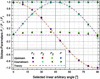Figure 3 Stokes–Poincare parameters P1, P2 and P3 from polarization measurements of light emitted by the downstream and upstream undulators at 375 eV. The polarization fraction P = (P1 2+P2 2+P3 2)1/2 is also shown. Comparison with the predicted values determined from simple theory indicates that the expected linear light emitted by both undulators is slightly elliptically polarized.

One explanation for these deviations is some misalignment of the undulator magnet arrays which would affect the calibration of the positions (gap and row phase) required to achieve a given photon energy and polarization angle. However, given that the observed contamination is extremely similar in light generated by both the upstream and downstream undulators, it would seem unlikely that the same misalignment is present in both undulators. Nevertheless, complete polarization measurements were carried out to observe how deliberately altering the phase of a single magnet array and the gap of the downstream undulator affects the beam polarization. The undulator gap and row phase were initially set to their nominal values (TRP/BRP = ±18.1325 mm, gap = 22.4934 mm) for LA45° from the calibrations tables, as discussed in §1. The TRP was then moved to several positions covering a range of ±0.2 mm about the nominal values while the BRP remained fixed. At each TRP the undulator gap was also altered, covering a range of −1.0 mm to +2.5 mm from the nominal gap. The beam polarization was determined for each of these configurations as shown in Fig. 4.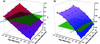Figure 4 The (a) P1 and (b) P3 Stokes–Poincaré parameters plotted as a function of row phase and gap offset from their nominal values at LA45° form surfaces which intersect the plane (green) of P1 = P3 = 0 (the expected values at LA45°). The best-fit lines of intersection (blue) for the two parameters cross at a common value of the row phase and gap offset which provides an undulator setting that should minimize both P1 and P3 simultaneously.

As expected, the Stokes–Poincaré parameters were shifted from their nominal values for different undulator configurations and it was observed that the P1 and P3 parameters (both expected to be zero at LA45°) were particularly sensitive to the changes. When plotted against the row phase and gap values it was found that both parameters approximate a plane which intersects the plane representing P1 = P3 = 0. A best-fit line of intersection between the two surfaces was determined for each parameter and the crossing point of the two lines provides a unique combination of gap and row phase which should minimize both parameters. This crossing point is found to lie at offsets of ΔTRP = +2.5 mm and Δgap = −0.6 mm. It is unrealistic that the magnet arrays could be misaligned by this margin since the laser tracker technology which was used to position the arrays with respect to external survey fiducials allows for positioning to a precision of better than 100 µm, and likely close to 50 µm. Further evidence is provided by simple analysis using theory describing the influence of undulator magnet arrays on photon beam polarization (Young et al., 2002). In this case we assume the circular contamination is the result of an effective phase shift of the magnetic field within the undulator. The horizontal (Bx) and vertical (By) components of the magnetic field along the central axis of an APPLE-II undulator are described by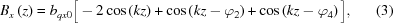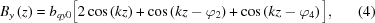where bqx0 and bqy0 are the magnitudes of the horizontal and vertical components of the on-axis field generated by a single magnet array, z is the position along the axis of the undulator,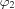and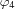are the row phase shifts of diagonally opposing magnet arrays (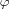= 2πΔz/λ) and k = 2π/λ with λ being the period of the magnet arrays. To generate linear light,andmove by equal and opposite distances, i.e.=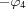=, which leads to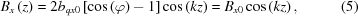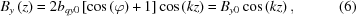so the magnetic field components Bx and By are in phase and the light is linearly polarized at an angle θ,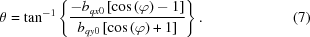However, if there is an error in one (or both) of the row phase positions, e.g.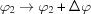, then the previous condition is broken, i.e.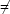. Consequently, Bx and By are no longer in phase and P3 becomes non-zero for any θ angles where P2 is non-zero, i.e. all angles other than linear horizontal and linear vertical (Koide et al., 1991). This leads to a behaviour of the P3 component which matches that observed in the complete polarization measurements: the magnitude increases to a maximum as the angle is increased from θ = 0°, reaching a maximum at θ = 45°, before falling again and reaching zero at θ = 90°. The maximum value of P3 observed is P3max = 0.075 which, in the theoretical description, corresponds to a row phase correction of ΔTRP = +0.8 mm. However, it has already been determined from the measurements above that ΔTRP = +2.5 mm would be required to minimize P3 for the I06 undulator. This indicates that, while it may be possible to minimize the P3 component by applying a (large) offset to the row phase position, the value of the offset differs considerably to that predicted by theory and points towards an additional influence on the polarization that increases the circular contamination of the linear light. A fundamental assumption of the above description is that the electron beam path is coincident with the central axis of the undulator magnet arrays. If in reality the electron beam is passing through the undulator slightly off-axis, this could have a significant impact on the photon beam polarization.

A series of measurements were carried out in cooperation with the accelerator physics group to investigate how the orbit of the electron beam through the I06 undulators impacts the beam polarization characteristics. Polarization analysis was performed using the nominal undulator configuration for LA45° (as above) with different combinations of horizontal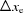and vertical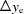displacements of the electron beam orbit (see Fig. 5a). Shifts of the electron orbit were achieved by altering the zero-point offsets of the beam position monitors before and after the undulator and allowing the corrector magnets, which work to maintain the electron beam orbit along a fixed path, to compensate for the change. Thus, the electron beam path is forced away from its nominal orbit in a stable and controllable manner.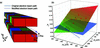Figure 5 (a) Measurements of the photon beam polarization were carried out for different horizontaland verticaloffsets of the electron beam orbit through the downstream undulator while the gap and row phase were set to produce LA45° light. (b) Similarly to the case where offsets in the top row phase and gap were introduced, when the P3 parameter is plotted against the offset coordinatesanda plane is formed which intersects the plane representing P3 = 0 and provides a set of configurations which minimize P3.

It was discovered that the P3 parameter is particularly sensitive to the electron orbit offset and, similarly to the previous alteration of the row phase and gap offset, the results were found to form a plane which intersects the plane representing the expected value of P3 = 0 (see Fig. 5b). In this case, the other Stokes–Poincaré parameters were insensitive to alteration of the electron beam path so no unique combination of horizontal and vertical beam offsets can be found to minimize P3. Instead, we find a set of solutions which lie along the intersection line. Following this, the electron beam was moved to several arbitrary coordinates described by this line and polarization measurements confirmed that they all minimize P3 as expected (P3 < 0.002 in all cases). A summary of these measurement results is shown in Table 1.

 Table 1Comparison of Stokes–Poincaré parameters measured at electron beam offset positions Δxe and Δye predicted to minimize P3 with those from the unshifted beam
 The column Index' refers to the corresponding numeric labels for the points in Fig. 5(b). Even in the worst case, the value of P3 is reduced by a factor of nearly 60 by shifting the electron beam.
Electron orbit offset
Index Δxe (µm) Δye (µm) P1 P2 P3
1 0 0 0.000 0.994 0.084
2 0 −260 0.000 1.001 0.000
3 −51 −235 −0.001 1.001 0.001
4 −92 −214 −0.003 1.007 0.001

The minumum offset required in either the horizontal or vertical directions required to find a solution is found to be at least ∼160 µm; however, it is unlikely that there is an error in the beam orbit of this magnitude. A more likely scenario would be that the undulator itself is slightly mispositioned by of the order of 100 µm. While the undulator magnet arrays are positioned with respect to the external mechanics to probably better than 100 µm as previously described, and the orbit of the electron beam itself is known to an extremely high degree of precision in its own reference frame, the relative co-alignment of these two coordinate systems is less certain. As such, it is not unreasonable to expect that there could be such a misalignment between the electron beam path and the undulator axis on the scale described here.

### 4. Conclusion

High-precision soft X-ray polarization analysis has been carried out using the Diamond polarimeter deployed at the I06 Nanoscience beamline at Diamond Light Source. Measurements of the linearly polarized beam at a range of arbitrary angles reveal a discrepancy between the observed and expected values for the Stokes–Poincaré parameters, specifically a non-zero P3 parameter which is expected to be zero for all linear polarizations. This behaviour, previously observed only in the downstream undulator (Hand et al., 2016), is now also confirmed to exist when using the upstream undulator under identical conditions. The possibility of row phase position mis-calibration was investigated by deliberately offsetting one of the magnet arrays, but the required mis­alignment of ΔTRP = +2.5 mm to correct P3 is judged to be unreasonably large for this to be the sole cause of the issue. Finally, a set of polarization measurements were carried out to test the impact of altering the electron beam orbit through the undulators. Similarly to the row phase and gap offset measurements, a set of electron beam offsets were found to correct the P3 discrepancy, but the minimum required orbit offset of ∼160 µm is rather large. However, given that both the row phase and electron beam offsets investigated here clearly contribute to some degree, it is likely that complete elimination of the circular component from the linearly polarized photon beam would ultimately require modification to both aspects.

These measurements extend our understanding of the mechanisms which contribute towards contamination of linear­ly polarized light by an unwanted circular component and help us quantify their impact on the final polarization state. The ultimate goal of this work is to systematically identify and correct all the underlying sources of polarization contamination within the beamline system, but due to limitations of time and resources an alternative approach (Bahrdt et al., 2010) may also be employed in the future to more quickly obtain the beam states required by users of the beamline. Nevertheless, it is already planned for additional simulations of the undulator sources to be carried out to better understand the contribution of an off-axis electron beam on the polarization contamination.

### Acknowledgements

This work was carried out with the support of Diamond Light Source Ltd (UK). The authors would like to thank Richard Mott, Andrew Malandain, Mark Booth, Jason Giles and Tony Lundyates for their technical assistance.

### References

This is an open-access article distributed under the terms of the Creative Commons Attribution (CC-BY) Licence, which permits unrestricted use, distribution, and reproduction in any medium, provided the original authors and source are cited.JOURNAL OFSYNCHROTRONRADIATION
ISSN: 1600-5775
Volume 25| Part 2| March 2018| Pages 378-384